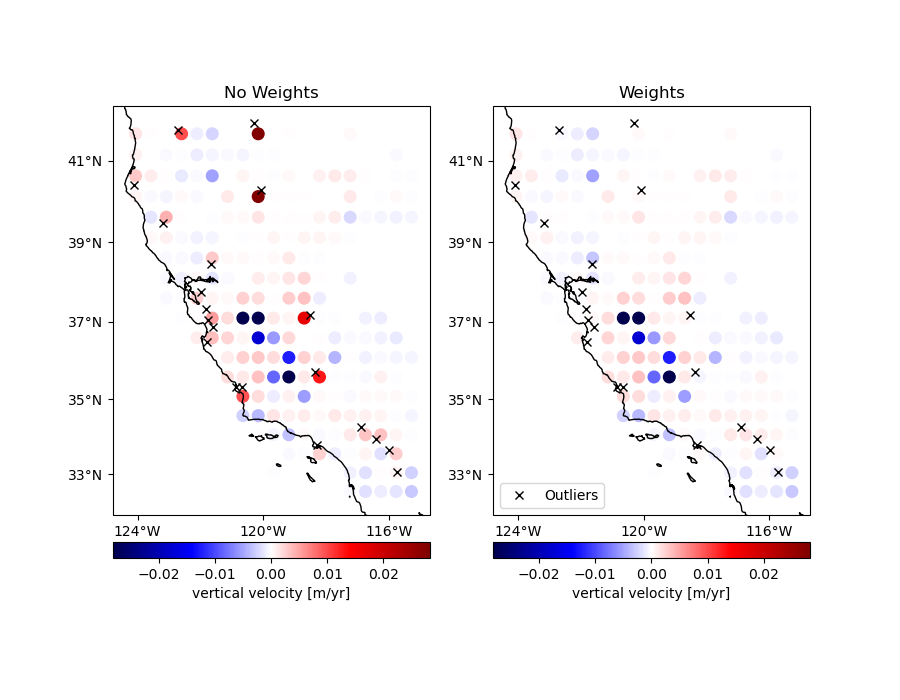# Using weights in blocked reduction¶

Sometimes data has outliers or less reliable points that might skew a blocked mean or even a median. If the reduction function can take a `weights` argument, like `numpy.average`, you can pass in weights to `verde.BlockReduce` to lower the influence of the offending data points. However, `verde.BlockReduce` can’t produce weights for the blocked data (for use by a gridder, for example). If you want to produced blocked weights as well, use `verde.BlockMean`.Out:

```Index of outliers: [1608 2347 1099 2408  674  433 1071 2452  124 1219 1126  938  875   51
2407  892  255 1349 1112 1326]
```

```import cartopy.crs as ccrs
import matplotlib.pyplot as plt
import numpy as np

import verde as vd

# We'll test this on the California vertical GPS velocity data
data = vd.datasets.fetch_california_gps()

# We'll add some random extreme outliers to the data
outliers = np.random.RandomState(2).randint(0, data.shape, size=20)
data.velocity_up[outliers] += 0.08
print("Index of outliers:", outliers)

# Create an array of weights and set the weights for the outliers to a very low
# value
weights = np.ones_like(data.velocity_up)
weights[outliers] = 1e-5

# Now we can block average the points with and without weights to compare the
# outputs.
reducer = vd.BlockReduce(reduction=np.average, spacing=30 / 60, center_coordinates=True)
coordinates, no_weights = reducer.filter(
(data.longitude, data.latitude), data.velocity_up
)
__, with_weights = reducer.filter(
(data.longitude, data.latitude), data.velocity_up, weights
)

# Now we can plot the data sets side by side on Mercator maps
fig, axes = plt.subplots(
1, 2, figsize=(9, 7), subplot_kw=dict(projection=ccrs.Mercator())
)
titles = ["No Weights", "Weights"]
crs = ccrs.PlateCarree()
maxabs = vd.maxabs(data.velocity_up)
for ax, title, velocity in zip(axes, titles, (no_weights, with_weights)):
ax.set_title(title)
# Plot the locations of the outliers
ax.plot(
data.longitude[outliers],
data.latitude[outliers],
"xk",
transform=crs,
label="Outliers",
)
# Plot the block means and saturate the colorbar a bit to better show the
# differences in the data.
pc = ax.scatter(
*coordinates,
c=velocity,
s=70,
transform=crs,
cmap="seismic",
vmin=-maxabs / 3,
vmax=maxabs / 3
)
cb = plt.colorbar(pc, ax=ax, orientation="horizontal", pad=0.05)
cb.set_label("vertical velocity [m/yr]")
vd.datasets.setup_california_gps_map(ax)
ax.legend(loc="lower left")
plt.show()
```

Total running time of the script: ( 0 minutes 0.917 seconds)

Gallery generated by Sphinx-Gallery